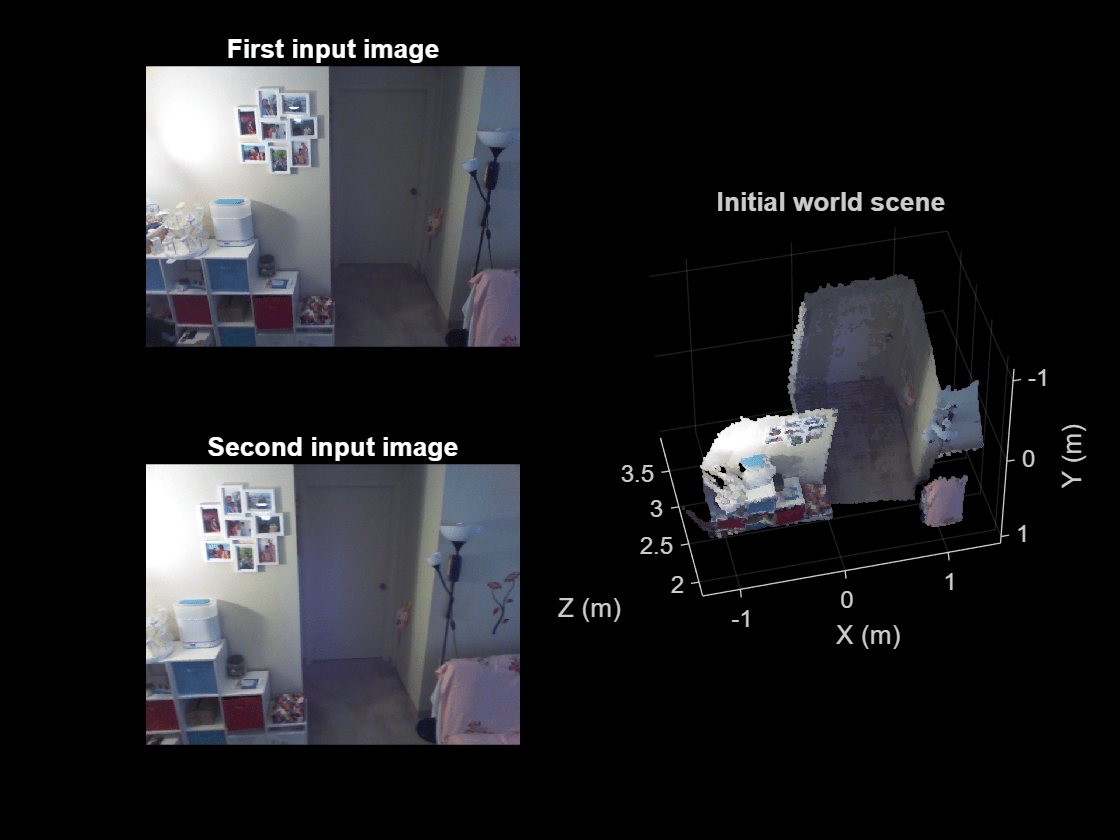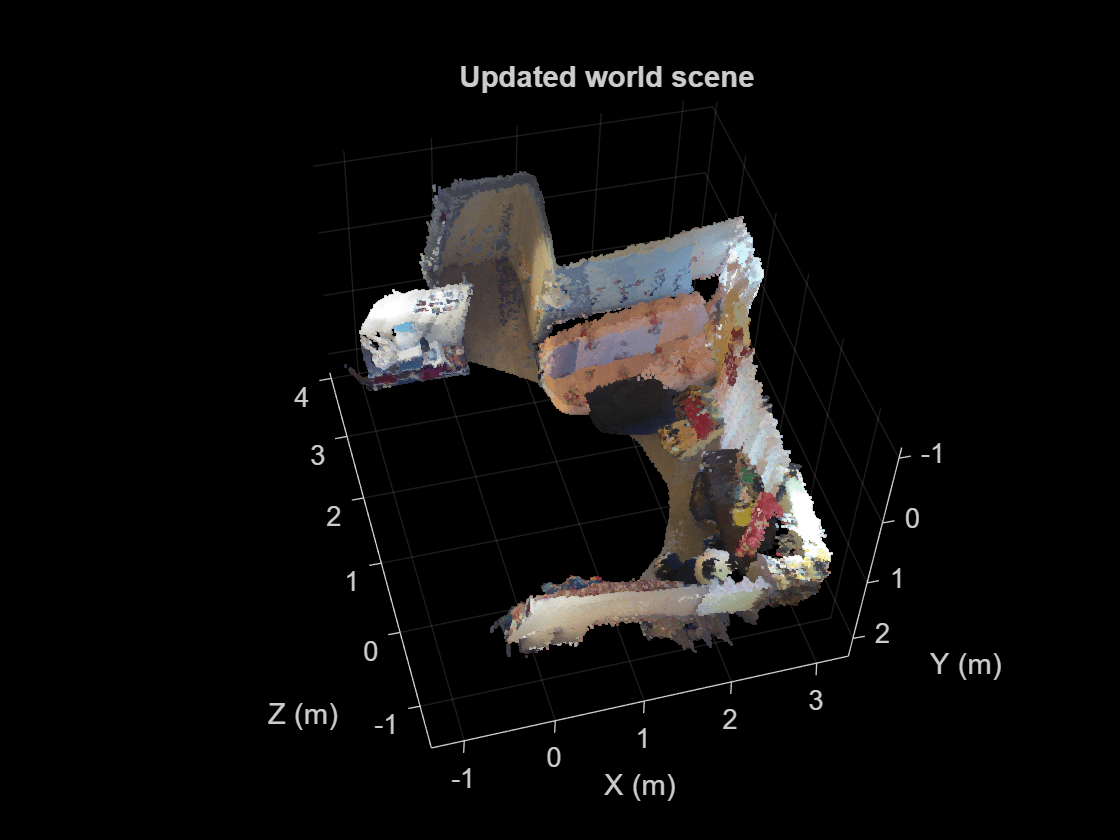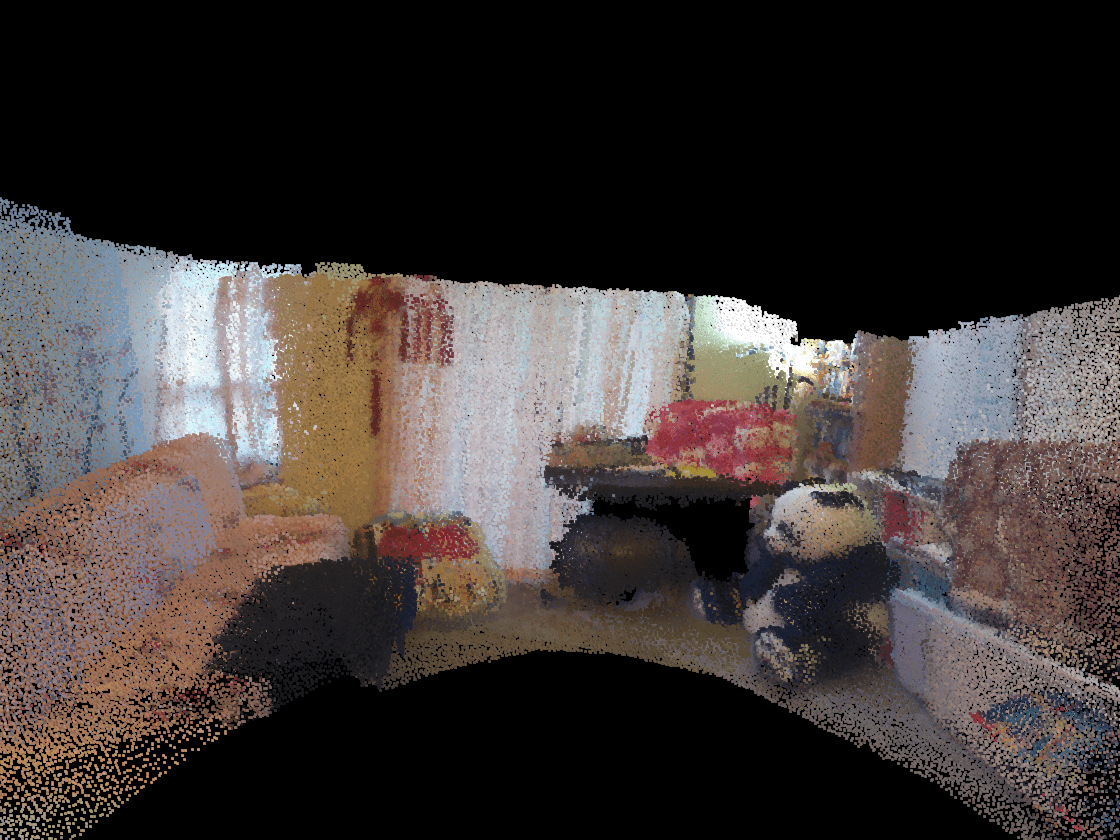# 3-D Point Cloud Registration and Stitching

This example shows how to combine multiple point clouds to reconstruct a 3-D scene using Iterative Closest Point (ICP) algorithm.

### Overview

This example stitches together a collection of point clouds that was captured with Kinect to construct a larger 3-D view of the scene. The example applies ICP to two successive point clouds. This type of reconstruction can be used to develop 3-D models of objects or build 3-D world maps for simultaneous localization and mapping (SLAM).

### Register Two Point Clouds

```dataFile = fullfile(toolboxdir('vision'), 'visiondata', 'livingRoom.mat'); load(dataFile); % Extract two consecutive point clouds and use the first point cloud as % reference. ptCloudRef = livingRoomData{1}; ptCloudCurrent = livingRoomData{2};```

The quality of registration depends on data noise and initial settings of the ICP algorithm. You can apply preprocessing steps to filter the noise or set initial property values appropriate for your data. Here, preprocess the data by downsampling with a box grid filter and set the size of grid filter to be 10cm. The grid filter divides the point cloud space into cubes. Points within each cube are combined into a single output point by averaging their X,Y,Z coordinates.

```gridSize = 0.1; fixed = pcdownsample(ptCloudRef, 'gridAverage', gridSize); moving = pcdownsample(ptCloudCurrent, 'gridAverage', gridSize); % Note that the downsampling step does not only speed up the registration, % but can also improve the accuracy.```

To align the two point clouds, we use the ICP algorithm to estimate the 3-D rigid transformation on the downsampled data. We use the first point cloud as the reference and then apply the estimated transformation to the original second point cloud. We need to merge the scene point cloud with the aligned point cloud to process the overlapped points.

Begin by finding the rigid transformation for aligning the second point cloud with the first point cloud. Use it to transform the second point cloud to the reference coordinate system defined by the first point cloud.

```tform = pcregistericp(moving, fixed, 'Metric','pointToPlane','Extrapolate', true); ptCloudAligned = pctransform(ptCloudCurrent,tform);```

We can now create the world scene with the registered data. The overlapped region is filtered using a 1.5cm box grid filter. Increase the merge size to reduce the storage requirement of the resulting scene point cloud, and decrease the merge size to increase the scene resolution.

```mergeSize = 0.015; ptCloudScene = pcmerge(ptCloudRef, ptCloudAligned, mergeSize); % Visualize the input images. figure subplot(2,2,1) imshow(ptCloudRef.Color) title('First input image','Color','w') drawnow subplot(2,2,3) imshow(ptCloudCurrent.Color) title('Second input image','Color','w') drawnow % Visualize the world scene. subplot(2,2,[2,4]) pcshow(ptCloudScene, 'VerticalAxis','Y', 'VerticalAxisDir', 'Down') title('Initial world scene') xlabel('X (m)') ylabel('Y (m)') zlabel('Z (m)')````drawnow`

### Stitch a Sequence of Point Clouds

To compose a larger 3-D scene, repeat the same procedure as above to process a sequence of point clouds. Use the first point cloud to establish the reference coordinate system. Transform each point cloud to the reference coordinate system. This transformation is a multiplication of pairwise transformations.

```% Store the transformation object that accumulates the transformation. accumTform = tform; figure hAxes = pcshow(ptCloudScene, 'VerticalAxis','Y', 'VerticalAxisDir', 'Down'); title('Updated world scene') % Set the axes property for faster rendering hAxes.CameraViewAngleMode = 'auto'; hScatter = hAxes.Children; for i = 3:length(livingRoomData) ptCloudCurrent = livingRoomData{i}; % Use previous moving point cloud as reference. fixed = moving; moving = pcdownsample(ptCloudCurrent, 'gridAverage', gridSize); % Apply ICP registration. tform = pcregistericp(moving, fixed, 'Metric','pointToPlane','Extrapolate', true); % Transform the current point cloud to the reference coordinate system % defined by the first point cloud. accumTform = affine3d(tform.T * accumTform.T); ptCloudAligned = pctransform(ptCloudCurrent, accumTform); % Update the world scene. ptCloudScene = pcmerge(ptCloudScene, ptCloudAligned, mergeSize); % Visualize the world scene. hScatter.XData = ptCloudScene.Location(:,1); hScatter.YData = ptCloudScene.Location(:,2); hScatter.ZData = ptCloudScene.Location(:,3); hScatter.CData = ptCloudScene.Color; drawnow('limitrate') end``````% During the recording, the Kinect was pointing downward. To visualize the % result more easily, let's transform the data so that the ground plane is % parallel to the X-Z plane. angle = -pi/10; A = [1,0,0,0;... 0, cos(angle), sin(angle), 0; ... 0, -sin(angle), cos(angle), 0; ... 0 0 0 1]; ptCloudScene = pctransform(ptCloudScene, affine3d(A)); pcshow(ptCloudScene, 'VerticalAxis','Y', 'VerticalAxisDir', 'Down', ... 'Parent', hAxes) title('Updated world scene') xlabel('X (m)') ylabel('Y (m)') zlabel('Z (m)')```